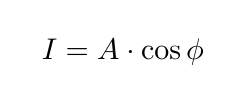# Syntax for maths in the forum

Not sure if it possible, but If it is easy to implement, a mathematical environment when writing a post would be welcome. Something like latex does when you begin by $and finish by$

$I = A \cdot \cos{\phi}$


which givesLots of post are requiring to put a bit of math but the forum itself isn’t very maths-friendly.

Quentin

That’s a good idea.
I searched for a plugin which supports it.
I found this one: https://meta.discourse.org/t/discourse-math-plugin/65770

@oana_hogoiu maybe we can install and activate it?

2 Likes

give it a try:

E=MC^2

E=MC^2

2 Likes

Nice! This renders
$$x = {-b \pm \sqrt{b^2-4ac} \over 2a}$$

to

x = {-b \pm \sqrt{b^2-4ac} \over 2a}

Thanks Florin.

Also inline is possible:

You know this: $a^2 + b^2 = c^2$


Is rendered to:
You know this: a^2 + b^2 = c^2

Some examples would be helpful. Does someone know a good page?
The MathJax docu is not so helpful

great - thank you!

Thanks a lot. It will make a lot of posts more readable.

Maybe I say something wrong but from the examples I observe here, the exact LaTex syntax is well understood

So I would advise to check out LaTex tutorials, which are very common

1 Like

That’s what I was looking for.
Thanks

For other people, here is probably the best reference I know for writing maths in LaTex format

1 Like